# 基于机器视觉的电磁阀表面缺陷检测技术研究Study on Surface Defect Inspection of an Electromagnetic Valve Based on Machine Vision

DOI: 10.12677/JISP.2020.91005, PDF, HTML, XML, 下载: 305  浏览: 464  科研立项经费支持

Abstract: Defect detection is mostly done by manpower. In the past, products were inspected to determine whether they were defective products by manual means, and the types of defects were recorded. A machine vision based solenoid valve defects inspection system was proposed in this work. This system is based on capturing images according to the different defects in the specimens, as well as inspection defects and frequency domains by using a CCD camera along with a machine vision algorithm and designs a lighting system. The inspection method first used an erosion and dilation algorithms of image morphology in the spatial domain. Mathematical logic operation was employed to retain the features of the image obtained by different algorithms. Although morphological image detection methods can quickly inspect defect contours, they have difficulty to inspect directional features i.e., thin scratches on specimen surfaces. Therefore, Fourier transform was applied to convert images from two-dimensional spatial domains as the frequency domain to inspect the defects that could not be inspected by the morphological method in the spatial domain. In the frequency domain, standard deviation was used. Therefore, defects were difficult to determine in the spatial domain, this paper uses Fourier transform to transform the image from the two-dimensional space domain to the frequency domain, and uses the Fourier spectrum to highlight the directional grain on the detection surface of the solenoid valve for surface defect detection. The experimental results show that defective images produce more noticeable differences in the Fourier spectrum. This difference is magnified using binary image processing, in which inverse Fourier transform transforms the Fourier spectrum into images in the spatial domain, and these images are compared. In this paper, the lighting system combining blue and white LED strip lights is used to inspect the defect of the solenoid valve in the space and frequency domains respectively.

1. 引言

2. 图像处理

3. 检测系统架构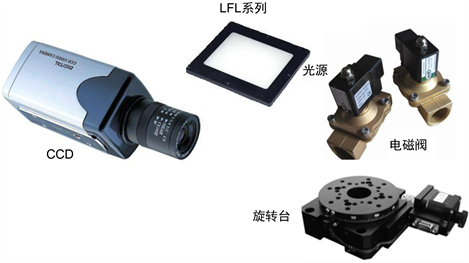(a) 面向光源系统组成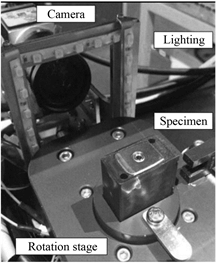(b) 实际系统架构

Figure 1. LED light source oriented system

4. 缺陷检测

4.1. 电磁阀的上盖卡榫检测方法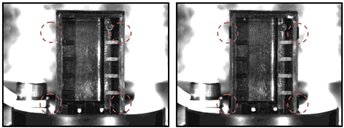Figure 2. Cogged defect detection of the originalFigure 3. Binarization image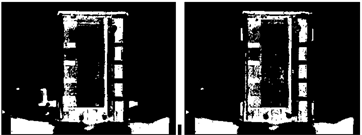Figure 4. Complementary color swap image processing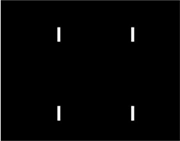Figure 5. Area of interest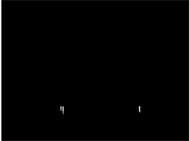(a) 缺陷结果：二个卡榫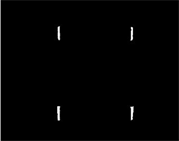(b) 正常结果：四个卡榫

Figure 6. Logic operation results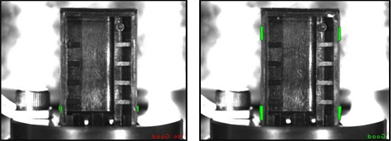Figure 7. Results of solenoid valve defect detection

4.2. 电磁阀的表面缺陷检测结果分析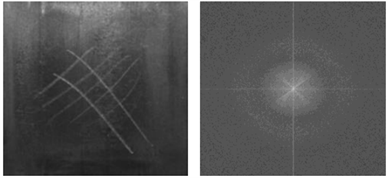Figure 8. Electromagnetic valve cogged defect detection results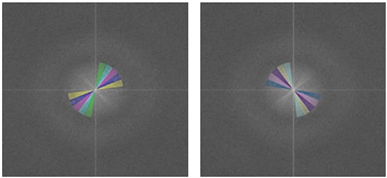Figure 9. Fourier spectrum partition map F~J

$\sigma =\sqrt{\frac{1}{N}{\underset{\theta ={\theta }_{1}}{\overset{{\theta }_{2}}{\sum }}\left({x}_{\theta }-\mu \right)}^{2}}$ (1)

${x}_{\theta }\text{=}\underset{R={R}_{1}}{\overset{{R}_{2}}{\sum }}\left\{G\left(r\mathrm{cos}\theta ,r\mathrm{sin}\theta \right)-{T}_{\mu }\right\}$ (2)

${T}_{\mu }=\frac{1}{A}\underset{0}{\overset{359}{\sum }}\underset{r={R}_{1}}{\overset{{R}_{2}}{\sum }}G\left(r\mathrm{cos}\theta ,r\mathrm{sin}\theta \right)$ (3)

$\mu =\frac{1}{N}\underset{\theta ={\theta }_{1}}{\overset{{\theta }_{2}}{\sum }}{x}_{\theta }$ (4)Figure 10. Fourier spectrum partition map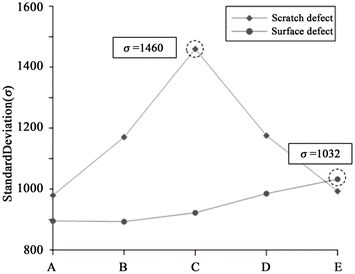(a) 频谱区间A~E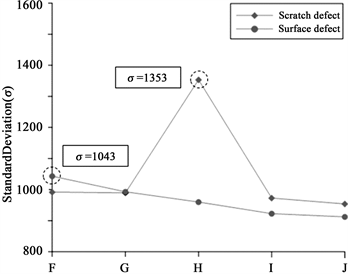(b) 频谱区间F~J

Figure 11. Standard deviation of spectrum interval grey value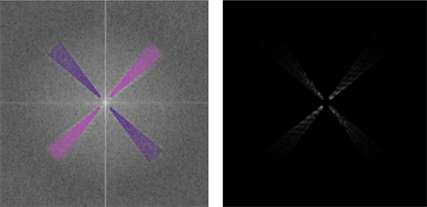Figure 12. Standard deviation higher C, interval H of keeping grey value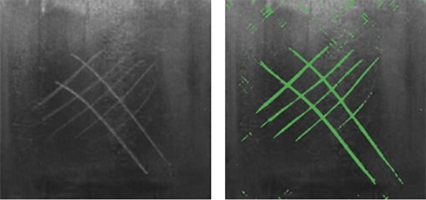Figure 13. Fourier reversal of interval C and H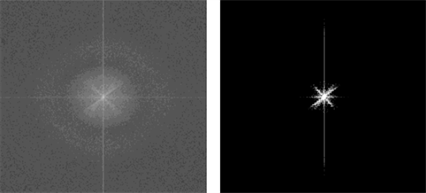Figure 14. Fourier spectrum for binary image processing results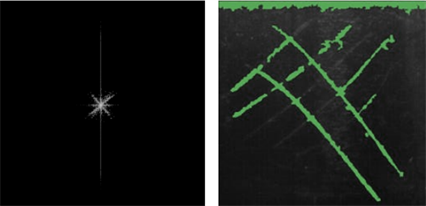Figure 15. Image is converted from frequency domain to space domain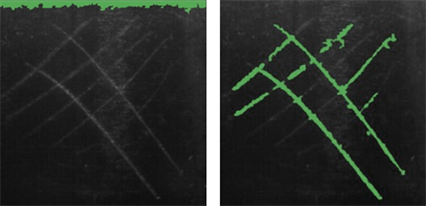Figure 16. Edge image processing results by noise elimination

5. 实验分析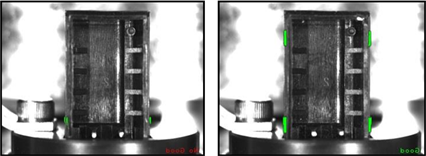Figure 17. Test results of solenoid valve defected

6. 结论

  吴永兴. 图像纹理旋转不变性分析[D]: [硕士学位论文]. 天津: 天津大学, 2007.  Chan, C.H. (2000) Fabric Defect Detection by Fourier Analysis. IEEE Transactions on Industry Applications, 36, 1267-1276. https://doi.org/10.1109/28.871274  Tsai, D.M. and Huang, T.Y. (2003) Automated Surface Inspection for Statistical Textures. Image and Vision Computing, 21, 307-323. https://doi.org/10.1016/S0262-8856(03)00007-6  王红梅, 张科, 李言俊. 基于小波变换的图像融合方法[J]. 红外与激光工程, 2005, 34(3): 79-83.  Elbehiery, H.M., Hefnawy, A.A. and Elewa, M.T. (2005) Visual Inspection for Fired Ceramic Tile’s Surface Defects Using Wavelet Analysis. ICGST International Journal on Graphics, Vision and Image Processing, 5, 67-74.  Zhang, X.W., Ding, Y.Q., Lv, Y.Y., Shi, A.Y. and Liang, R.Y. (2011) A Vision Inspection System for the Surface Defects of Strongly Reflected Metal Based on Multi-Class SVM. Expert Systems with Applications, 38, 5930-5939. https://doi.org/10.1016/j.eswa.2010.11.030  李胜军, 郑灼. 基于显微成像与图像处理的工件表面漏光缺陷检测算法[J]. 组合机床与自动化加工技术, 2017(6): 141-144.  汤勃, 孔建益, 伍世虔. 机器视觉表面缺陷检测综述[J]. 中国图象图形学报, 2017, 22(12): 1640-1663.  梁雄, 张舞杰, 李听听. 一种基于Blob分析的摄像头模组缺陷检测方法[J]. 电子设计工程, 2015(15): 19-22.  徐江涛, 姚素英, 朱天成. CMOS有源像素传感器光响应分析及实验模型建立[J]. 传感技术学报, 2007, 20(2): 334-337.  Clarke, F.A.D. (2010) Modelling Visual Search for Surface Defects. Heriot-Watt University, Edinburgh.  沈乔楠, 安雪晖. 基于游程递归的连通区域标记算法[J]. 计算机应用, 2010, 30(6): 1616-1618.  Suzuki, K., Horiba, I. and Sugie, N. (2003) Linear-Time Connected-Component Labeling Based on Sequential Local Operations. Computer Vision & Image Understanding, 89, 1-23. https://doi.org/10.1016/S1077-3142(02)00030-9  He, L.F., Chao, Y.Y. and Suzuki, K. (2007) A Run-Based Two-Scan Labeling Algorithm. IEEE Transactions on Image Processing, 17, 749-756. https://doi.org/10.1109/TIP.2008.919369  徐利华, 陈早生. 二值图像中的游程编码区域标记[J]. 光电工程, 2004, 31(6): 63-65.  Diaz-de-Leon, J.L. and Sossa-Azuela, J.H. (1996) On the Computation of the Euler Number of a Binary Object. Pattern Recognition, 29, 471-476. https://doi.org/10.1016/0031-3203(95)00098-4  Sossa-Azuela, J.H., Santiago-Montero, R., Perez-Cisneros, M., et al. (2013) Computing the Euler Number of a Binary Image Based on a Vertex Codification. Journal of Applied Research & Technology, 11, 360-369. https://doi.org/10.1016/S1665-6423(13)71546-3  林小竹, 籍俊伟, 谷莹莹. 图像欧拉数的研究与应用[J]. 石油化工高等学校学报, 2007, 20(2): 88-91.  He, L.F. and Chao, Y.Y. (2015) A Very Fast Algorithm for Simultaneously Performing Connected-Component Labeling and Euler Number Computing. IEEE Transactions on Image Processing, 24, 2725-2735. https://doi.org/10.1109/TIP.2015.2425540  冈萨雷斯, 伍兹. 数字图像处理[M]. 阮秋琦, 阮宇智, 译. 北京: 电子工业出版社, 2011.  Liu, Z., Wang, W. and Wang, P. (2010) Design of Machine Vision System for Inspection of Rail Surface Defects. Journal of Electronic Measurement & Instrument, 24, 1012-1017. https://doi.org/10.3724/SP.J.1187.2010.01012  Wang, Z.-Y. (2013) Research on Steel Plate Surface Defects Detection Method Based on Machine Vision. Computer & Modernization, 7, 97-117.  Li, X.K., Gao, C., Guo, Y.C., et al. (2013) The Inspection Method Based on Distributed Machine Vision for Surface Defects of Bridge Cables. Optical Technique, 39, 424-428.  Liu, B., Wu, S.-J. and Zou, S.F. (2009) Automatic Detection Technology of Surface Defects on Plastic Products Based on Machine Vision. International Conference on Mechanic Automation and Control Engineering, Wuhan, 26-28 June 2010, Vol. 37, 2213-2216.